Blog

360dissertations understands how tough Masters level projects can be. The standard of a post graduate course is higher than a bachelor’s degree.

Ordinal logistic regression, an extension of simple logistic regression test, is a statistical technique used to predict the relationship the relationship between an ordinal dependent variable and one or more independent variables. Commonly known as ordinal regression test, this statistical technique lets you determine if the independent variables have statistical significant effect on the dependent variables.

Examples,

A researcher investigates the factors that influence the decision of applying to the post graduate degree. Students were questioned if they are very likely, likely or somewhat likely to apply to the post graduate degree. The outcome of the research has three variables. (1) the institution is private or public, (2) present GPA and (3) educational status of the parents. The researcher believed that the difference between likely, somewhat likely and very likely are not equal.

Ordinal regression can be used to determine the reaction of patients to drug dosage. The outcomes can be classified as severe, moderate, mild or none. Here, it was believed that the difference between mild and moderate is not easy to quantify and the difference between mild, moderate, severe is not equal.

Performing ordinal regression involves checking for data and ensuring they hold good for all the assumptions that are needed to obtain a valid result. The assumptions of ordinal logistic regression model are as follows.

• The dependent variable must be measured at an ordinal level.

• One or more independent variables are ordinal, categorical or continuous. The ordinal independent variables must be treated as either continuous or categorical and not as an ordinal variable (when performing the test in SPSS). Examples of categorical variable include ethnicity, gender, profession and so on. Examples of continuous variables include age, income, revision time, weight, intelligence, etc.

• There exists no multicollinearity. When two or more independent variables are highly correlated with each other, multicollinearity occurs. This contributes to difficulty in understanding technique problems in conducting the test and determining which variable result in explanation of the dependent variable.

• There exists proportional odd. This assumption means that the independent variable has identical effect at the cumulative split of the dependent variable.

Conducting ordinal regression in SPSS

The ordinal regression in SPSS can be performed using two approaches: GENLIN and PLUM. Although GENLIN is easy to perform, it requires advanced SPSS module. Therefore, PLUM method is often used in conducting this test in SPSS.

1. Converting log odds to log ratio - PLUM procedure doesn’t produce confidence intervals or odds ratio. Instead it generates log odds. However, it can be converted into odds ratio by using output management system (OMG) control panel.

1. The next step is to run the PLUM procedure. This procedure produces results such as predicted probabilities. The PLUM can be performed by

• Clicking analyse -> regression -> ordinal

• Transfer the dependent variable to the dependent box, categorical independent variables to factors box, continuous independent variables to covariate box

• Click options -> continue to return to the ordinal regression dialogue box

• Click output & select the necessary options. Click continue to return to the ordinal regression dialogue box

• Click on location button to specify the regression model.

• Click on the paste button to open syntax editor, place the suitable code at the end of the syntax, then click run -> all to generate output

1. After running the PLUM procedure, output the file consisting of parameter estimates table information using the OMG panel.

• Click utilities -> OMS control panel to open the OMG panel and view the prior selected requested

• Click on end all button to change the ‘status’ column ‘end’ in the request box

• Click on OK to view the OMG panel summary and click OK once again to exist

1. After producing the output in the output viewer window and new SPSS data file, the file needs to be saved.

• Click file -> save as and save the file

1.  Generate odds ratio and 95% confidence intervals.

• Click file -> new -> syntax and choose the right dataset

• Copy the highlighted syntax into the syntax editor and calculate the odds ratio

• Click run -> all to generate the output

After running the test and generating the output, the next step is to interpret the results. The stages involved here are:

1. Analyse multi linear regression that was ran to test for multicollinearity

2. Check whether the regression model includes overall goodness-of-fit

3. Determine if the independent variables have statistical significance on the dependent variables

4. For categorical independent variables, interpret that the odds of one group has a higher or lower value on the dependent variable

5. For continuous independent variable, interpret how decrease and increase in that variable

6. Identify how the ordinal regression model predicts the dependent variable

Note - if the proportional odds assumption is violated, one should consider using multinomial logistic regression.

Big data refers to dealing with a huge amount of raw data that can be gathered, stored and analyzed to determine the pattern or trend pertaining to the data. As per the data experts by 2020, about 40 trillion gigabytes of data. Although obtaining an analytical picture of data is important, the hard reality is focusing on specific details among the availability of a vast amount of raw data is easier said than done. This is when big data visualization comes into the picture.

Big data visualization is the process of transforming analyzed data into a readable visual format. This is achieved by presenting the data in the form of charts, graphs, tables, and other forms of visuals. With the growing need for presenting the data in readable formats, data visualization tools have gained importance than ever. Some of the popular big data visualization tools that one must look forward to are:

1. Kemal Density estimation for non-parametric Data:- Non-parametric data is nothing but a form of data in which the information pertaining to the population and the underlying distribution of data is unknown. This form of information can be visualized using the Kernel density function. The Kernel density function represents the probability distribution of random variables. This technique is generally used when the parametric distribution of data doesn’t add sense and random data assumptions need to be avoided.

2. Box & Whisker plot for huge data:- A binned box with Whiskers represents the outliers and distribution of the large data. This technique includes five statistics such as minimum, lower quartile, median, upper quartile and maximum to summarize the distribution of data set. The upper quartile will be represented by the upper edge of the box, on the other hand, the lower quartile is described by the lower edge of the box. The minimum & maximum value are represented by Whiskers, and the median is defined by a central line that segregates into sections.

3. Network diagrams & word clouds:- Semistructured and unstructured data require unique visualization techniques. Word cloud technique, often used on unstructured data, demonstrates the frequency (high or low) of a word within the body of text with its size in the cloud. On the other hand, network diagrams can be used on both semistructured and unstructured data. Here, the relationships (individual actors within the network) are represented as nodes and ties (relationships between individuals).

4. Correlation matrices:- This technique combines big data and fast response times and lets you determine the relationships between the variables. The correlation matrix includes a table to display the correlation coefficient between variables. Each cell will demonstrate t relationship between the two variables. A correlation matrix can also be used to summarize the data, as a diagnostic for advanced analysis and as an input to further advanced analysis.

5. Line charts:- Also known as the row graph, line charts are the simplest form of data visualization technique that can be used for big data as well as traditional data. This type of chart includes a graph of information represented using a number of rows. The line chart plots the dependency or relationship of one variable on another.

6. Heat maps:- A heat map is a form of data visualization technique which represents the displayed information in two dimensions by color values. This technique provides an instant overview of the data pattern. Heat maps or thermal maps can be of various types. However, note that all the maps will transmit interactions between information values and use different colors that are difficult to comprehend.

7. Histogram plot:- A histogram plot is one of the commonly used data visualization techniques in the big data field. This technique represents the distribution of continuous variables over a given period or interval of time. Histogram plots the data by dividing it into small intervals known as bins. This plot can also be used to investigate the underlying distribution, skewness, frequency, outliers, and many more.

To conclude, consider the requirements of your study prior to choosing the data visualization technique. This is because if the data pattern is not identified and isn’t represented accurately, then the data collected will not add value to your study.

Most often, repeated measures ANOVA test is the first choice among the researchers for determining the difference between 3 or more variables. However, if assumptions such as normal distribution isn’t met, an alternative test known as Friedman test is used.

Friedman test, an extended version of one-way ANOVA with repeated measures, is a non-parametric test used to determine the difference between 3 or more matched or paired groups. Basic ANOVA test has the assumptions of a normal distribution with their corresponding variances, but Friedman test eliminates these assumptions of normal distribution. The method incorporates ranking for each block together afterwards analyzing the values of ranks in each column. The Friedman test is primarily a 2-way ANOVA used for the data that is Non - parametric.

In Friedman test one variable serves as a treatment/group variable and another as a blocking variable. Here, the dependent variable must be continuous (but not normally distributed) and independent variable must be categorical (time/condition)

Like any other statistical test, this test too consists of a few assumptions including:

• The samples should not be normally distributed

• There is one group that is measured on three or more occasions

• The dependent variable must be measured at an ordinal or continuous level

• A group is a random sample from the population

Prior to conducting this test, a researcher must set up hypothesis such as:

• Null hypothesis - It states that the medians of values of each group are equal. Simply put, the treatments have no effect

• Alternative hypothesis - The medians of values of each group are not equal indicating that there is a difference between the treatments

Today, although several tools such as SPSS, SAS, etc. are used to perform Friedman test, the most tool among the researchers is R language.

So, how do you conduct this test in R?

In order to conduct this test in R, import the file into R and refer to the variables directly within the data set. This is followed by analysing the data using the command “Friedman.test.” Create a matrix or table, fill the data and run the test using the “Friedman test ()” command.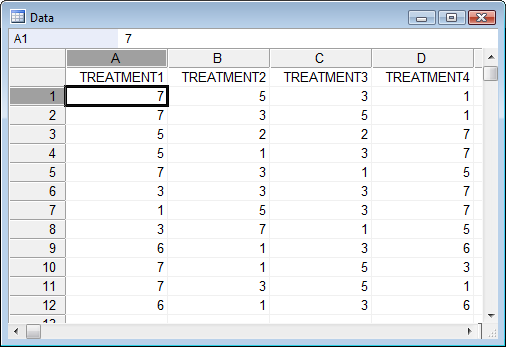Upon completion of the analysis, the next step is to interpret the results. I.e. to check if the test is statistically significant or not. To accomplish this, compare the P value with the significance level.

However, before interpreting the results, it should be noted that the Friedman test ranks values in each row. As a result, the test is not affected by sources of variability that equally affect all values in a row. Typically, a significance level of 0.05 works well. We check the value at 5 % significance level.

• P-value ≤ significance level : If the p-value less than significance level, then we can reject the idea that the difference between the columns are the result of random sampling, concluding that at least one column differs from another.

•  P-value > significance level : If the P value is greater than the significance level, then the data doesn’t provide you with significant evidence to conclude that overall medians differ. However, this isn’t similar to stating that all medians are similar.

Although the outcome of Friedman tells you if the groups are significantly different from each other, they do not tell you which groups differ from each other. This is when post hoc analysis for the Friedman’s test comes into the picture.

The primary goal here is to investigate which all pairs of groups are significantly different from each other. If you have N groups, to check all of their pairs, you will have to perform n^2 comparisons, therefore the need of correcting multiple comparisons arise.

The initial step in a post hoc analysis in R to find out which groups are responsible for the rejection of the null hypothesis. For simple ANOVA test, there exists a readily available package that can directly calculate post hoc analysis -TukeyHSD.

This is followed by understanding the outputs from the test run. In the case of simple ANOVA, a box plot would be sufficient, but in the case of repeated measure test, a boxplot approach can be misleading. Therefore you can consider using two plots: (a) one for parallel coordinates (b) other boxplots of the differences between all pairs.

Glimpse at an example for Friedman test

Consider an experiment where 6 persons (block) received 6 different diuretics (groups) that were A to F. Here the response determines the concentration of Na in human urine and the observations were recorded after each treatment.

> require(PMCMR) [library used to perform Friedman test]

> r <- matrix(c(

+ 3.88, 5.44, 8.96,8 .25, 4.91, 12.33, 28.58, 31.14, 16.92,

+ 24.19, 26.84, 10.91, 25.24, 39.52, 25.45, 16.85, 20.45,

+ 28.67, 4.44, 7.94, 4.04, 4.4, 4.23, 4.36, 29.41, 37.72,

+ 39.92, 28.23, 28.35, 12, 38.87, 35.12, 39.15, 28.06, 38.23,

+ 26.65),

Nrow = 6,

Ncol = 6,

+ dimnames = list (1:,c("a”,"b","g","h","i","j")))

> print (r)

a b g h i j 1 3.88 5.4 48.96 8 .25 4.91 12.33 28.58 31.14 16.92,

24.19 26.8 10.91 25.2 39.5 25.45 16.85 20.45

28.67 4.4 47.94 4.04 4.4 4.23 4.3629.41 37.72

39.9228.23 28.35 12 38.87 35.12 39.15 28.06 38.23

26.65

STS > friedman .test(y)

Friedman chi-squared (χ 2) = 23.333,

Degree of freedom = 5 , p-value = 0.000287

Result -  using friedman test

χ 2 (5) = 23.3- chi square test

p < 0.01

Note:

A different post hoc tests can be performed by using the command posthoc.friedman.conover.test in the PMCMR package.

Research often involves circumstances where the assumption of normality is violated. This is when a statistical test such as logistic regression involving non-normal variables come handy. Logistic regression, known as the logit model, is an analytical method to examine/predict the direct relationship within an independent variable and dependent variables. It is used to model binary result variables. Today, this statistical technique is considered to be one of the most commonly used methods to predict a real-valued result.

Logistic regression is a particular case of the generalized linear model where the dependent variable or response variable is either a 0 or 1. It returns the target variables as probability values. However, we can transform and obtain the benefits using threshold value. It should be noted that logistic regression considers only the probability of the dependent variable and uses Generalized Linear Model (GLM).

To successfully perform logistic regression test a few assumptions need to be considered. This includes:

The dependent or response variable should be binomially distributed.

There should be a linear relationship between the independent variables and the logit or link function.

The response variable must be mutually exclusive.

Typically, logistic regression can be calculated using equations like,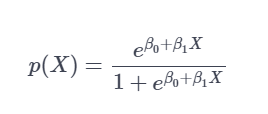The above equation can be transformed as: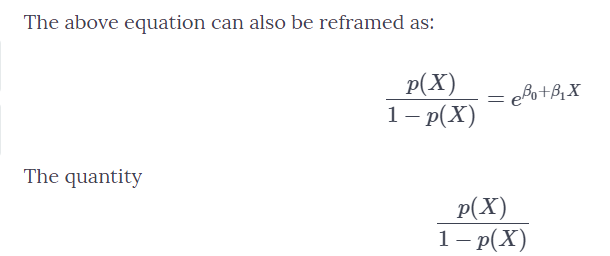Taking log on both sides the final equation can be written as :Although statistical tools such as python, sklearn, statsmodel, etc. can be used to perform this test, R language is one tool that is the right-fit for performing logistic regression. This is because R is open-source software, which has a wide variety of libraries and packages available to perform this statistical test with ease. However, prior to conducting the test, it is a must to clean the data, failing to which the output obtained may be inaccurate.

Steps involved in performing logistic regression in R language

Consider an example of binary logistic regression which is analysed using ISLR package.

Step 1: This step involves installing and loading the ISLR package. To do so, use the data sets present in the package and then apply the model on real data set.

Note: names() is used to check the names & data in the data frame

head() is a glimpse of the first few rows.

summary() is a function used for getting all the major insights of the data.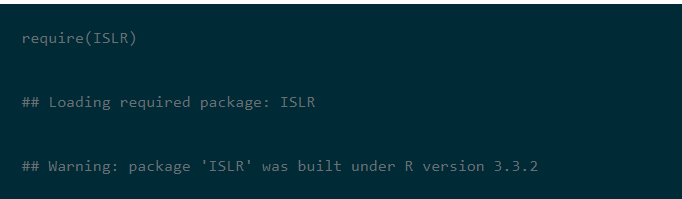The density box gives an insight into the separation of Up and Down. It creates an overlapping picture in direction value. Also, GLM () function is used to implement generalized linear models.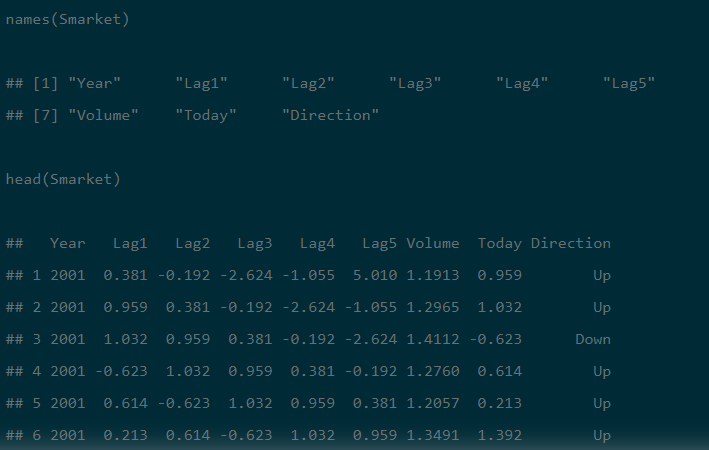Step 2:  The library  “CARET ” should be installed and then used to list down all the variables. This is followed by treating the outliers, missing values and create new variables using feature engineering technique and then train the sample.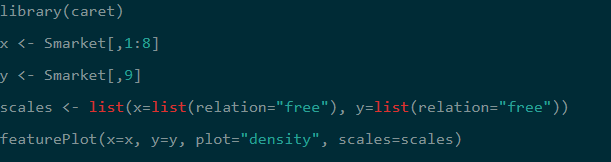Step 3: Here the summary() has to be returned to all the estimates, standard errors, z-score, and p-values for every coefficient. In the below example, it is observed that none of the coefficients are significant. This step gives us the null deviance and the residual deviance, with a minimal difference of 2 & 6 degrees of freedom.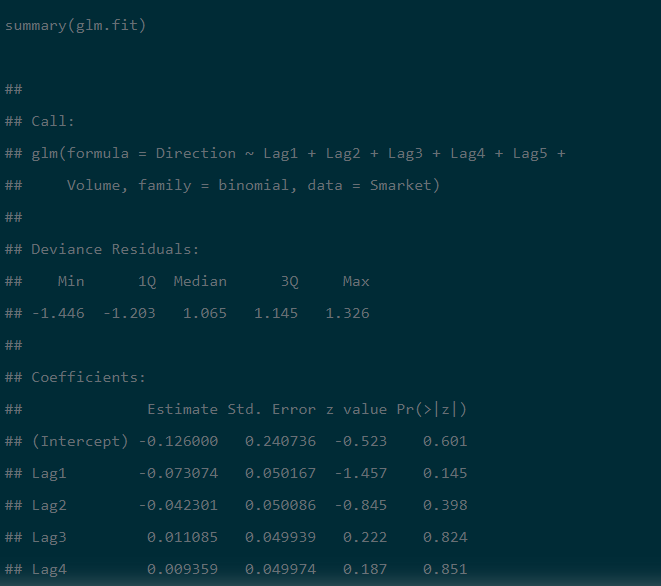Step 4: In the fourth step, training and test the samples. To make the process easier, segregate the sample into two trains and then test the sample.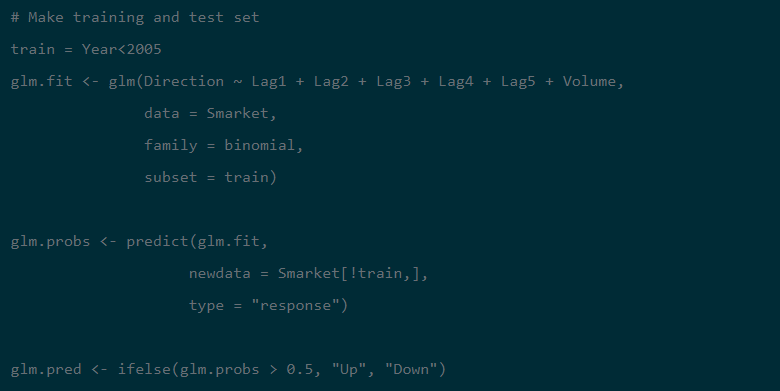STEP 5: Use the function Predict2 <- predict (Smarket, type=’response’) to predict the pending data.

STEP 6: Check the predictability and reliability of the model using ROC Curve

Note: ROC is an area under the curve which gives us the accuracy of the model. It is also known as the index of accuracy which represents the performance of the model.

To interpret, the greater the area under the curve, the better is the model.

Graphically it can be represented using two axis. Where one is true positive rate and another represents false positive rate. If the value approaches to 1 then better the model .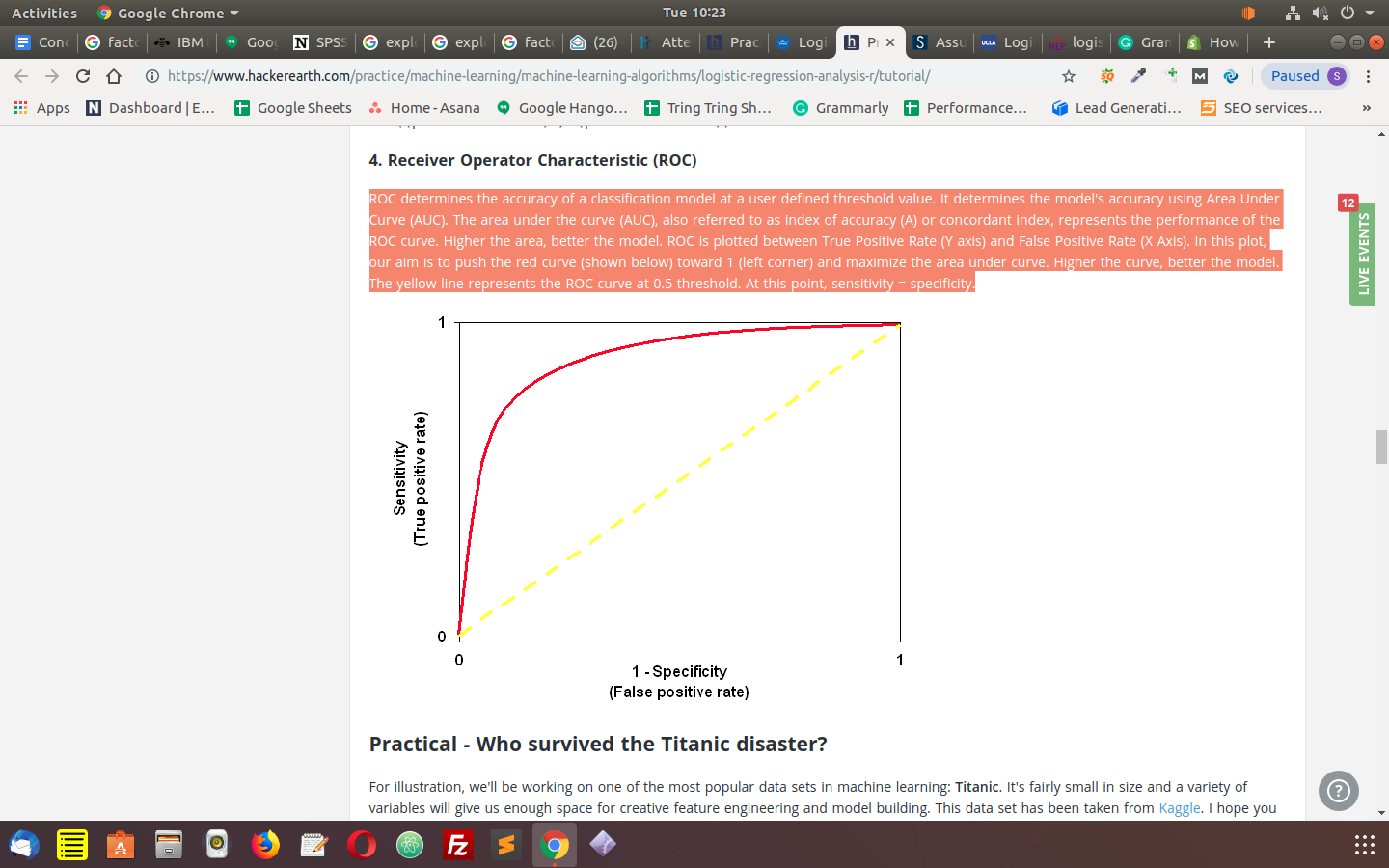The commands used to check the predictability are:

library(ROCR)

ROCRpred1 <- prediction(predict1, train\$Recommend)

ROCRperf1 <- performance(ROCRpred1, 'tr','fr')

plot(ROCRperf1, colorize = TRUE )

Now, plot glm function using ggplot2 library

library(ggplot2)

ggplot(train, aes(x=Rating, y=Recommend)) + geom_point() +

stat_smooth1(method="glm", family="binomial", se=FALSE)

GLM () model does not assume that there is any linear relationship between independent or dependent variables. Nevertheless, it does assume a linear relationship between link function and independent variables in the logistic model.

In the above example, a 59% classification rate is achieved. This indicates that a smaller model perform betters.

Data occupies the ultimate position in the research process. A research procedure involves collection, analysis and interpretation of data. In the current era, one can easily collect plenty of information from various sources. However, not all data gathered will be useful and relevant to a study. One should thoroughly inspect and decide which information would help them in conducting their study. This is when the data mining process comes into the picture.

Data mining process involves filtering and analysing the data. It uses various tools to identify patterns and relationships in the information, which is then used to make valid predictions.

This process involves several techniques such as clustering, association, classification, prediction, decision tree, sequential pattern techniques have been developed which are widely used in various fields.

To conduct data ming process without any hassle, plenty of tools are available in the market. Among them, the most popular software trusted by the research community include:

Weka - This machine learning tool, also called as Waikato Environment, is built at the University of Waikato in New Zealand. Written in the JAVA programming language, this software supports significant data mining tasks such as data processing, visualisation, regression, and many more. Additionally, Weka is best suited for data analysis as well as predictive modeling. It consists of algorithms, visualisation software that support the machine learning process and operates on the assumption that data is available in the form of a flat-file. Weka has a GUI to give easy access to all its features in addition to SQL Databases via database connectivity.

Orange - This component based software aids data mining and visualisation process. Written in Python computing language, its components are known as ‘widgets’. The widgets range from data visualisation, preprocessing to the evaluation of algorithms and predictive modeling. The widgets offer characteristics such as presenting data table & enabling to choose features, reading the data, comparing learning algorithms, etc. Data in Orange gets formatted to the desired pattern swiftly and can be easily moved by simply flipping/moving the widgets. It also allows the user to make smarter decisions by comparing & analyzing the data.

KNIME - This tool is considered as the best integration platform for data analytics. Operating on the theme of the modular data pipeline, KNIME uses the assembly of nodes to preprocess the data for analytics & visualisation process. It constitutes different data mining and machine learning components embedded together. This tool is popularly used by the researchers for performing a study in the pharmaceutical field. KNIME includes some excellent characteristics, such as quick deployment and scaling efficiency. Additionally, predictive analysis is made accessible to even naive users.

Sisense - Considered as the best suited BI tool, Sisense has the potential to manage and process small as well as a large amount of data. Designed specially for non-technical users, this software enables widgets as well as drag & drop features. Sisense produces reports that are highly visual and lets combining data from different sources to develop a common repository. Further various widgets can be selected to develop the reports in the form of line charts, pie charts, bar graphs, etc. based on the purpose of a study. Reports can be drilled down merely by clicking to investigate details and comprehensive data.

DataMelt - DataMelt, also called as DMelt is a visualisation and computation environment offering an interactive framework to perform data mining and visualisation. DMelt is written in JAVA programming language, is designed mainly for technical users and for the science community. It is a multi-platform utility and can work on any operating system that is compatible with Java Virtual Machine (JVM). DMelt consists of scientific libraries to produce 2D/3D plots and mathematical libraries to develop curve fitting, random numbers, algorithms, etc. This software can also be utilised for analysis of large data volumes or statistical analysis.

SAS data mining - SAS or Statistical Analysis System is developed by SAS Institute for the purpose of analytics & data management. This tool can mine data, modify it, and handle data from various sources and conduct statistical analysis. It allows the user to analyse big data and derives precise insight to make timely decisions. SAS offers a graphical UI for non-technical users and is well suited for text mining, data mining, & optimisation. The added advantage of this tool is that it has a highly scalable distributed memory processing architecture.

IBM SPSS modeler - Owned by IBM, this software suite is used for data mining & text analytics to develop predictive models. IBM SPSS modeler consists of a visual interface that lets the user to work with data mining algorithms without any need for programming. It offers additional features such as text analytics, entity analytics etc. and removes the unnecessary hardships faced during the data transformation process. It also allows the user to access structured as well as unstructured data and makes it easy for them to use predictive models.

Data mining tools are important to leverage the existing data. Adopt trusted & relevant tools, use them to the fullest potential, uncover hidden patterns & relationships in data and make an impact for your research.